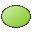# KR Card

This card creates a mesh of surface triangles in the shape of circular region with or without a hole. It is also possible to create an elliptical region.

On the Construct tab, in the Surfaces group, click theEllipse (KR) icon.

## Parameters:

S1
The centre point of the circle.
S2
A point that is located at any distance perpendicular to and above the plane of the circle.
S3
A point on the outer arc.
S4
If there is a value present for this parameter, then a circular ring is created. S4 must be located between S1 and S3.
The angle $\phi$ (degrees)
The angle subtended by the arc in degrees.
Maximum edge length (outer)
The maximum edge length of the triangles along the outer edge of the arc in m (is scaled by the SF card). If this parameter is left empty, the value specified with the IP card is used.
Maximum edge length (inner)
When a disk with a hole is created, this parameter defines the maximum edge length for the triangles along the inner edge of the arc in m (is scaled by the SF card). If this parameter is left empty, the value specified with the IP card is used.
Scale second half axis with
If this parameter is empty or is set to 1, a circular disk is created. If set to $\frac{\text{b}}{a}$ , an elliptical disk is created. Here $\frac{\text{b}}{a}$ gives the ratio of the two half axes, where a is the distance S1–S3. It is recommended to generate elliptical disks with extremely small or extremely large axial ratios with a CAD system as the distortion formulation used in PREFEKO may fail in these cases
The circle’s plane is perpendicular to the line S1–S2. This length is arbitrary. The radius of the disc is given by the length between the points S3 and S1. The area that is to be subdivided (the shaded region in the figure for the KR card dialog) is generated by sweeping the edge S3–S1 around the axis S1–S2 through $\phi$ degrees in the mathematically positive sense. For $\phi$ = 360° a circle is obtained.

The fineness of the mesh is determined by the maximum edge length specified by the last IP card prior to the KR card. Along the arcs, accurate modelling of the geometry may require finer segmentation and the maximum edge length values specify the maximum edge length along the outer and inner (if applicable) arcs respectively. If any of these values are not specified the length specified with the IP card will be used on the corresponding arc.

The normal vectors of the triangles on the disk all point in the direction from S1 to S2.

## Examples of the KR card usage

The following example meshes of a circular plate, a flat circular ring and a flat elliptical ring are all created using the KR card.